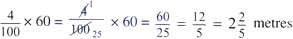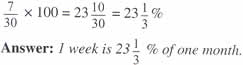Tuesday , July 5 2022# NCERT 5th Class (CBSE) Mathematics: Percentage

## FINDING PERCENTAGE

#### A. Finding the percentage as a number

(a) Arjun’s mother bought a pair of shoes for him at a sale. The shoes coast Rs. 300, but she got it at 25% less than that. How much money did she save?

We need to find 25% of Rs. 300

Step 1. Write the per cent as a fraction: 25/100

Step 2. Multiply by the quantity: 25/100 × 300 = 75

Answer: Arjun’s mother saved Rs. 75.

(b) What is 4% of 60 meters?#### B. Finding What per cent one number is of another

(a) Ankita got Rs. 225 from making and selling Diwali card during the school fete. She spent Rs. 90 on buying a present at the fete for her mother. What percentage of her money did she spend?

To find what per cent 90 is of 225:

Step 1. Express 90 out of 225 as a fraction → 90/225

Step 2. Change the fraction into a percentage by multiplying by 100 → 90/225 ×  100 = 40%

Answer: Ankita spent 40% of what she earned on buying a present for her mother.

(b) What per cent is 50 g of 1 kg?

Step 1. Make both the units same → 1 kg = 1000 g

Step 2. Express 50 g out of 1000 g as a fraction → 50/1000

Step 3.Change the fraction to a percentage by multiplying by 100 → 50/1000 × 100 = 5%

Answer: 50 g is 5% 0 1 kg

(c) What per cent is 1 week of 1 month?

(Take one month to have 30 days.)

1 week = 7 days

1 month = 30 daysCHECKPOINT: Use the same units while finding percentages.

## चुनौती हिमालय की 5th NCERT CBSE Hindi Rimjhim Ch 18

चुनौती हिमालय की 5th Class NCERT CBSE Hindi Book Rimjhim Chapter 18 प्रश्न: लद्दाख जम्मू-कश्मीर राज्य में …